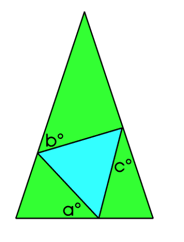### Submit a Resource

NRICH: Terminology

Given an equilateral triangle inside an isosceles triangle, can you find a relationship between the angles?

The green triangle is an isosceles triangle while the blue triangle is an equilateral triangle.Find a in terms of b and c.

What can you say about the triangles if a=b=c?

Age 14 to 16

Math Topics
Algebra & Pre-Algebra, Equations, Geometry
High School, Educator

## Organization

NRICH (University of Cambridge)

Challenge